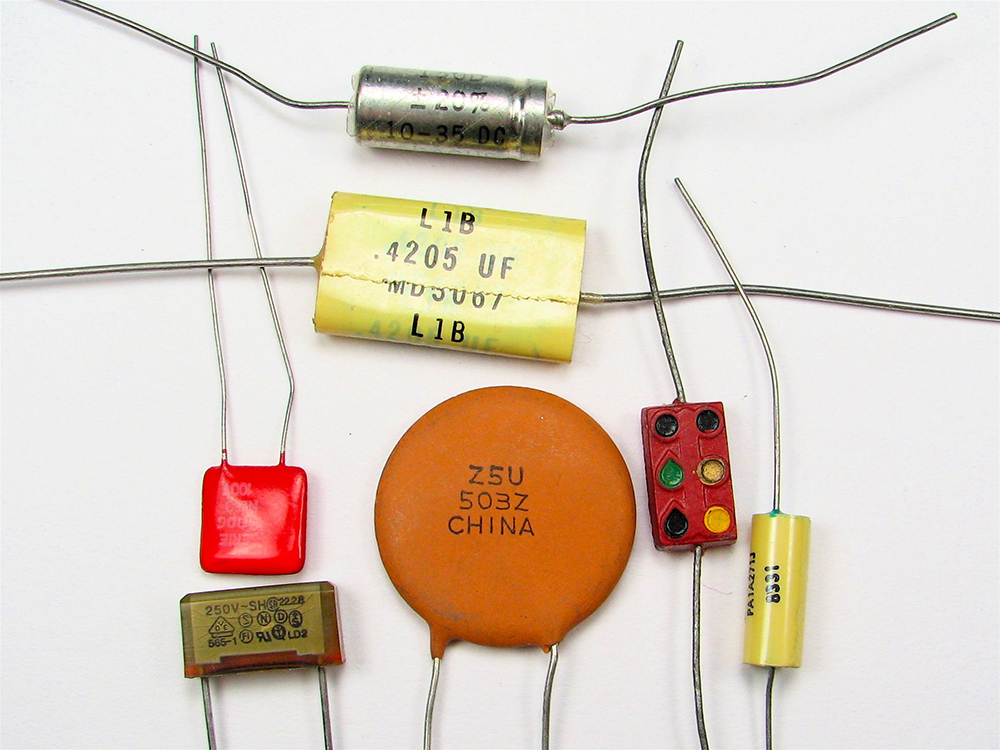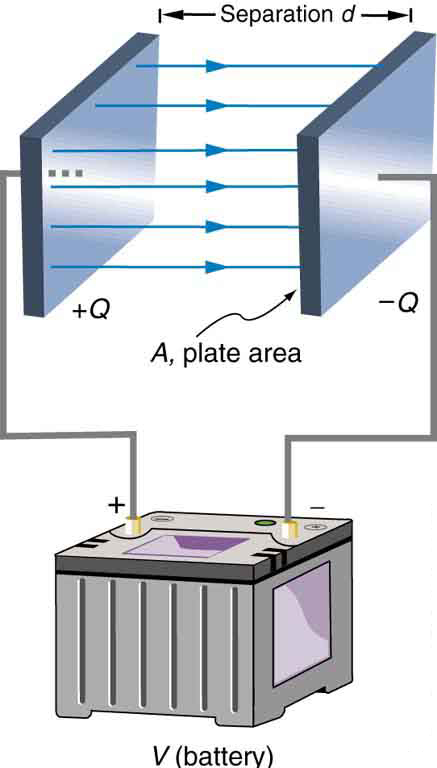# 19.5 Capacitors and dielectrics  (Page 2/12)

 Page 2 / 12
$Q=\text{CV}.$

This equation expresses the two major factors affecting the amount of charge stored. Those factors are the physical characteristics of the capacitor, $C$ , and the voltage, $V$ . Rearranging the equation, we see that capacitance $C$ is the amount of charge stored per volt, or

$C=\frac{Q}{V}.$

## Capacitance

Capacitance $C$ is the amount of charge stored per volt, or

$C=\frac{Q}{V}.$

The unit of capacitance is the farad (F), named for Michael Faraday (1791–1867), an English scientist who contributed to the fields of electromagnetism and electrochemistry. Since capacitance is charge per unit voltage, we see that a farad is a coulomb per volt, or

$\text{1 F}=\frac{\text{1 C}}{\text{1 V}}.$

A 1-farad capacitor would be able to store 1 coulomb (a very large amount of charge) with the application of only 1 volt. One farad is, thus, a very large capacitance. Typical capacitors range from fractions of a picofarad $\left(1 pF={\text{10}}^{\text{–12}}\phantom{\rule{0.25em}{0ex}}\text{F}\right)$ to millifarads $\left(1 mF={\text{10}}^{–3}\phantom{\rule{0.25em}{0ex}}\text{F}\right)$ .

[link] shows some common capacitors. Capacitors are primarily made of ceramic, glass, or plastic, depending upon purpose and size. Insulating materials, called dielectrics, are commonly used in their construction, as discussed below.Some typical capacitors. Size and value of capacitance are not necessarily related. (credit: Windell Oskay)

## Parallel plate capacitor

The parallel plate capacitor shown in [link] has two identical conducting plates, each having a surface area $A$ , separated by a distance $d$ (with no material between the plates). When a voltage $V$ is applied to the capacitor, it stores a charge $Q$ , as shown. We can see how its capacitance depends on $A$ and $d$ by considering the characteristics of the Coulomb force. We know that like charges repel, unlike charges attract, and the force between charges decreases with distance. So it seems quite reasonable that the bigger the plates are, the more charge they can store—because the charges can spread out more. Thus $C$ should be greater for larger $A$ . Similarly, the closer the plates are together, the greater the attraction of the opposite charges on them. So $C$ should be greater for smaller $d$ .Parallel plate capacitor with plates separated by a distance d size 12{d} {} . Each plate has an area A size 12{A} {} .

It can be shown that for a parallel plate capacitor there are only two factors ( $A$ and $d$ ) that affect its capacitance $C$ . The capacitance of a parallel plate capacitor in equation form is given by

$C={\epsilon }_{0}\frac{A}{d}.$

## Capacitance of a parallel plate capacitor

$C={\epsilon }_{0}\frac{A}{d}$

$A$ is the area of one plate in square meters, and $d$ is the distance between the plates in meters. The constant ${\epsilon }_{0}$ is the permittivity of free space; its numerical value in SI units is ${\epsilon }_{0}=8.85×{\text{10}}^{–\text{12}}\phantom{\rule{0.25em}{0ex}}\text{F/m}$ . The units of F/m are equivalent to ${C}^{2}\text{/N}·{\text{m}}^{2}$ . The small numerical value of ${\epsilon }_{0}$ is related to the large size of the farad. A parallel plate capacitor must have a large area to have a capacitance approaching a farad. (Note that the above equation is valid when the parallel plates are separated by air or free space. When another material is placed between the plates, the equation is modified, as discussed below.)

## Capacitance and charge stored in a parallel plate capacitor

(a) What is the capacitance of a parallel plate capacitor with metal plates, each of area $\text{1.00}\phantom{\rule{0.25em}{0ex}}{\text{m}}^{2}$ , separated by 1.00 mm? (b) What charge is stored in this capacitor if a voltage of $3.00×{\text{10}}^{\text{3}}\phantom{\rule{0.25em}{0ex}}\text{V}$ is applied to it?

Strategy

Finding the capacitance $C$ is a straightforward application of the equation $C={\epsilon }_{0}A/d$ . Once $C$ is found, the charge stored can be found using the equation $Q=\text{CV}$ .

Solution for (a)

Entering the given values into the equation for the capacitance of a parallel plate capacitor yields

$\begin{array}{lll}C& =& {\epsilon }_{0}\frac{A}{d}=\left(8.85×{\text{10}}^{\text{–12}}\frac{F}{m}\right)\phantom{\rule{0.10em}{0ex}}\frac{1.00\phantom{\rule{0.25em}{0ex}}{\text{m}}^{2}}{1.00×{\text{10}}^{–3}\phantom{\rule{0.25em}{0ex}}\text{m}}\\ & =& 8.85×{\text{10}}^{–9}\phantom{\rule{0.25em}{0ex}}\text{F}=8.85 nF.\end{array}$

Discussion for (a)

This small value for the capacitance indicates how difficult it is to make a device with a large capacitance. Special techniques help, such as using very large area thin foils placed close together.

Solution for (b)

The charge stored in any capacitor is given by the equation $Q=\text{CV}$ . Entering the known values into this equation gives

$\begin{array}{lll}Q& =& \text{CV}=\left(8.85×{\text{10}}^{–9}\phantom{\rule{0.25em}{0ex}}\text{F}\right)\left(3.00×{\text{10}}^{3}\phantom{\rule{0.25em}{0ex}}\text{V}\right)\\ & =& \text{26.6 μC.}\end{array}$

Discussion for (b)

This charge is only slightly greater than those found in typical static electricity. Since air breaks down at about $3\text{.}\text{00}×{\text{10}}^{6}\phantom{\rule{0.25em}{0ex}}\text{V/m}$ , more charge cannot be stored on this capacitor by increasing the voltage.

#### Questions & Answers

a15kg powerexerted by the foresafter 3second
Firdos Reply
what is displacement
Xolani Reply
movement in a direction
Jason
hello
Hosea
Explain why magnetic damping might not be effective on an object made of several thin conducting layers separated by insulation? can someone please explain this i need it for my final exam
anas Reply
Hi
saeid
hi
Yimam
What is thê principle behind movement of thê taps control
Oluwakayode Reply
while
Hosea
what is atomic mass
thomas Reply
this is the mass of an atom of an element in ratio with the mass of carbon-atom
Chukwuka
show me how to get the accuracies of the values of the resistors for the two circuits i.e for series and parallel sides
Jesuovie Reply
Explain why it is difficult to have an ideal machine in real life situations.
Isaac Reply
tell me
Promise
what's the s . i unit for couple?
Promise
its s.i unit is Nm
Covenant
Force×perpendicular distance N×m=Nm
Oluwakayode
İt iş diffucult to have idêal machine because of FRİCTİON definitely reduce thê efficiency
Oluwakayode
if the classica theory of specific heat is valid,what would be the thermal energy of one kmol of copper at the debye temperature (for copper is 340k)
Zaharadeen Reply
can i get all formulas of physics
BPH Reply
yes
haider
what affects fluid
Doreen Reply
pressure
Oluwakayode
Dimension for force MLT-2
Promise Reply
what is the dimensions of Force?
Osueke Reply
how do you calculate the 5% uncertainty of 4cm?
melia Reply
4cm/100×5= 0.2cm
haider
how do you calculate the 5% absolute uncertainty of a 200g mass?
melia Reply
= 200g±(5%)10g
haider
use the 10g as the uncertainty?
melia
which topic u discussing about?
haider
topic of question?
haider
the relationship between the applied force and the deflection
melia
sorry wrong question i meant the 5% uncertainty of 4cm?
melia
its 0.2 cm or 2mm
haider
thank you
melia
Hello group...
Chioma
hi
haider
well hello there
sean
hi
Noks
hii
Chibueze
10g
Olokuntoye
0.2m
Olokuntoye
hi guys
thomas
the meaning of phrase in physics
Chovwe Reply
is the meaning of phrase in physics
Chovwe

### Read also:

#### Get Jobilize Job Search Mobile App in your pocket Now!

Source:  OpenStax, College physics. OpenStax CNX. Jul 27, 2015 Download for free at http://legacy.cnx.org/content/col11406/1.9
Google Play and the Google Play logo are trademarks of Google Inc.

Notification Switch

Would you like to follow the 'College physics' conversation and receive update notifications?ByBy Vongkol HENGBy Alec MoffitBy Nicole DuquetteBy Mucho MizindukoBy Rylee MinllicBy Sebastian Sieczko...By Yasser IbrahimBy Madison ChristianBy OpenStaxBy Rhodes